# Formula Interpreter 1 - Introduction¶

The formula interpreter can be used to calculate new channels and add them to a given NC file. Example: If a file contains the channels speed and torque, a new channel power =(speed * torque) / 9549.3 can be calculated. If speed or torque values are edited, dependent channels can be updated.

If data of the speed or torque channel is modified, the formula interpreter can update the dependent channels.

For MDF-, BLF-, Famos- or ASAM-ODS-Data the formula can contain channels with different number of data points. In this case the formula interpreter will interpolate all used channels to the time base of the channel with the highest number of data points.

For all other data files the number of data points of all channel used in a formula must contain the identical number of data points. The result of the formula is a channel the the same number of data points. Global file attributes can be used in the formula, for example displacement = 2000. If a formula does not contain any channels the formula channel will be added to the channel group of the fist channel in the NC file (Channel with dimid=0).

## An Example¶

• Import the data file test1.xls from the directory c:\Program Files\UniPlot\Samples. Click on the >> button to fully open the data browser: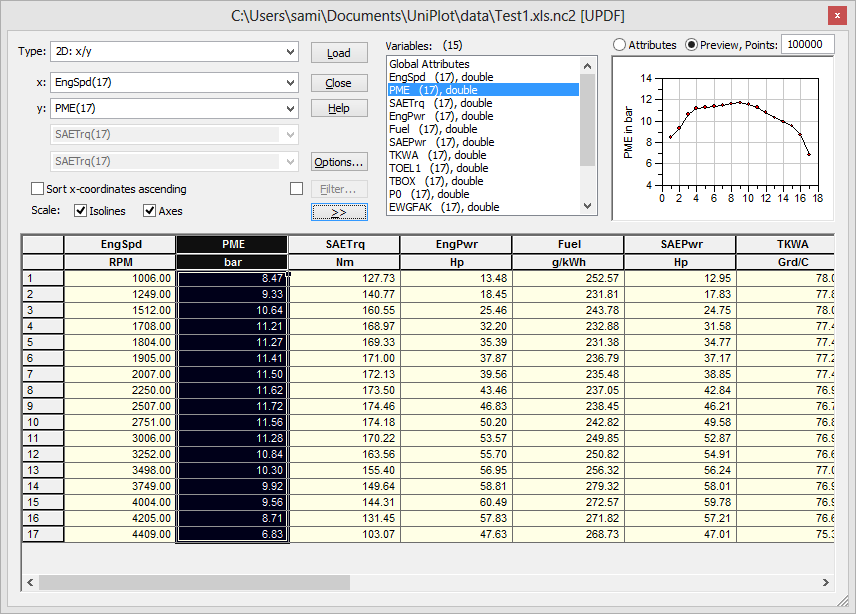• Right click on a channel name in the data grid and choose the command Add Formula Channel to open the following dialog box: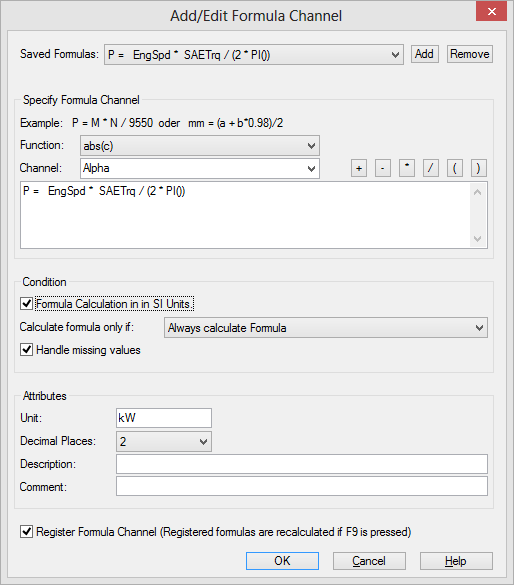Type the following formula into the text field:

Power = (EngSpd * SAETrq)/9550

The formula creates the channel Power.

• Click OK to add the channel to the NC file.

## The Add Formula Channel Dialog Box¶Saved Formulas
This combo box lists the last 128 formulas. To add a formula to the text field, click the Add button.
Functions

Lists all functions which can be used in the formula editor dialog box. To add a function to the text field, select a formula from the list.

A list with all predefined functions can be found in Functions in Formulas. This chapter also explains how to add user defined functions.

Channel

This field displays a list with channel names. To add a channel to the text field, select a name from the list.

The list displays global attributes and constant values as well.

Operators
The operators +, -, *, / can be used in a formula. Click the operator button to add the operator to the text field.

Formula Text Field

Specify the formula in this text field. A formula has the following structure:

NewChannel = Expression


The formula starts with the channel name followed by the assignment Operator = followed by the formula expression. You can use blank characters anywhere between operators, identifiers, and constants.

A channel name that already exists in the NC file will be overwritten.

Formula calculation in SI Units

If this option is checked, all channels used in the formula will be converted to SI units before the formula is evaluated. The formula result is converted into the specified unit. The calculation is only possible if all units are specified in the formula table, see Unit Converter.
Calculate formula only if
You can specify conditions to decide if a formula should be calculated or not, e.g diesel, turbo etc. If the condition is not true, the formula will not be calculated. See Definition of a Condition.
Handle Missing Values
This option should normally be checked. If you would like to handle missing values in the formula yourself, clear this option.
Unit
Specify the channel unit.
Decimal Places
Specify the number of decimal places for the new channel.
Description
The description is displayed in the formula list, see Tools=>Formulalist.
Comment
Here, you can type a comment.
Register Formula

If the option is not selected, the formula will only be evaluated once. The formula will be saved in the NC file. To edit the formula, right click on the formula channel in the data grid and select Add Formula Channel.

When this option is marked, the formula will be registered and evaluated every time F9 is pressed.

The formula is saved as an IC file in the formula directory. The file name is the name of the formula channel with the prefix _fi_ and the file name extension .ic, e.g. _fi_Power.ic.

The formula is saved in the directory that is specified in the Formula Configuration dialog box.

All formulas in the directory are saved in one library file. The file name is formula.icl9 and the file is saved in the formula directory.

OK
When OK is clicked, the formula is checked and evaluated. If the formula contains a syntax error or unknown channel names, an error message is generated. In this case fix the problem and click OK again.

## Defining Formulas¶

The formula can contain the following elements:

• Parentheses (). The number of parentheses levels is not limited.

• The +, -, *, /, ** operators are supported. The power operator can be used instead of **. The character ^ can be used as a power operator. Attention: Instead of ** and ^ it is recommended to use the pow() function, because it handles missing values. (see Functions in Formulas).

• Numbers of the following form; 1.23, 1e12, -13. Only the period (.) is allowed as the decimal character (no comma).

• Names of measured channels or other formula channels. The name must begin with an alphabetic character an underscore (‘_’), followed by zero or more alphanumeric characters including the underscore. Case is significant.

Beginning with UniPlot R2013.11 formulas can be defined with placeholders for similar channels. Example: For each cylinder of an engine, the cylinder pressure p_cyl_1 to p_cyl_4 is measured. Instead of defining a formula for each measurement point name, a formula with a placeholder for each cylinder can be defined. For example, the variable name for the formula could be p_cyl_<c>. The measurement point identifier is <c>. The result channel must contain the same identifier, for example: p_cyl<c>_smooth:

p_cyl<c>_smooth = smooth(p_cyl_<c>)


A variable name can contain up to 3 differnt placeholders. A placeholder starts with < followed by one to three small letters followed by >. Measurement point identifiers with one letter replace the values 1 to 9. Two letters replace the values 01 to 99. Three letters replace the values 001 to 999. Measurement point identifier should be separated by a letter or underscore. Example: T<c>_b<b>. c could be the cylinder number and b the bank of a V8 engine.

The identifier must occur in the result channel:

p_<c>_<i> = T<i> * X<c>


Channel names with measurement point identifier can also be used in the units table. See Channel Sheet.

• Function calls, for example abs() (see Functions in Formulas).

• The string length of a formula is limited to 255 characters including blank characters.

• Constants can be used inside a formula. The constants should be defined in the constant table (see The Constant Table).

Valid formulas are e.g.:

a = B_20 * Rho_20 * 1000 / P_EWG
b = c_percent / 100


while the following formula is invalid:

p = (n_Motor * Md_Motor) / 9550,0


The decimal separator is the point. The number 9550,0 is not permitted.

x = 100 * B_20 / V-ACT


The character "-" is not permitted in the channel name.

• From R2013.12 formulas with intermediate results can be entered:

a1 = M1+M2
a2 = M1+M3
M_Result = a1 * a2


The formula generates the channel M_Result. For the intermediate result a1 and a2 no channel are created. Because each formula is a separate line, the end of each sub formul is the newline character.

If the calculation of the intermediate result require multiple lines, each formula must be terminated with a semicolon. In this case each sub formula must be terminated with a semicolon. Even the formulas that fit in one line. Example:

a1 = M1+
M2;
a2 = M1+M3;
M_Result = a1 *
a2;


## Alternative Formula¶

If different formulas have to be used to calculate a formula channel, e.g. different measurement equipment is unsed in the test cells, alternative formulas can be specified.

Two or more alternative formulas can be defined as following:

Use the formula editor The Add Formula Channel Dialog Box to define the formulas. Use different names for the formula result, for eample add _a, _b, etc. to the channel name (Example: Tcalc_a, Tcalc_b, Tcalc_c). The formulas will be saved in the formula directory. Open these files with UniPlot and rename the result channel from Tcalc_a to Tcalc, Tcalc_b to Tcalc etc.

In the following example the names are already modified from Tcalc_a to Tcalc (first element in the return value and first parameter in set_ch):

def _fi_Tcalc_a(bInfo)
{
ssChannels = "TOEL1, SB";
if (bInfo) {
return ["Tcalc"; ..                      // <----
"Â°C"; ..
""; ..
ssChannels; ..
""; ..
"%.2lf"];
}
_fich_Tcalc_a = ch("TOEL1", "TOEL1");
_fich_SB = ch("SB", "TOEL1");
_fich_T_Calc = _fich_Tcalc_a .* _fich_SB;

set_ch("Tcalc", _fich_Tcalc_a, ssChannels);       // <----
}


Save the files and choose the command Tools=>Update formula.

## Functions in Formulas¶

Formulas specified in an Excel table can contain function calls such as sqrt( 98.15 / (T_Intake + 273.15) ) which calculates the square root of 98.15 / (T_Intake + 273.15).

Here is a list of the specified functions:

### abs(c)¶

Calculates the absolute value of c.

### and(c1, c2)¶

Boolean AND operator. Is used mainly in conjunction with the if_than_else function.

### fft_filter(time, c, rsFreqCutOffBelow, rsFreqCutOffAbove)¶

The time channel unit must be seconds.

If the time frequence is not constant the data is resampled to a constant frequence. For the result an FFT is calculated. The freqences above and below the given values are set to 0. An iFFT is calculted and interpolted to the given frequency.

### atan(c)¶

Calculates the arcus tangents of c.

### between(c, lower, upper)¶

c greater or equal lower and c smaller or equal upper. Is used mainly in conjunction with the if_than_else function.

### bit(c, nBit)¶

Returns the Bit nBit of channel c. nBit starts with 1.

### log(c)¶

log calculates the natural logarithm of c. The natural logarithm has the irrational number e (≈ 2.718) as its base.

### log10(c)¶

calculates the base-10 logarithm of c.

### cos(c)¶

Calculates the cosinus of c.

### tan(c)¶

Calculates the tangents of c.

### counter()¶

Creates a list of valus 1, 2, 3, ... for the first dimension.

### counter(ssChannelname)¶

Creates a channel with the values 1, 2, 3, .. . The channel will be added to the same dimension as the given channel ssCahnnelname.

### exp(c)¶

Calculates the exponentia function e^c, where e is Euler’s number. (approximately 2.718281828)

### int(c)¶

Cuts off the decimal places of channel c. 2.7 will be cut to 2.0.

### find_plateau(ctime, cData, dy_tolerance, min_len, max_len, y_min_threshold, y_maxn_threshold)¶

Finds plateaus in the data.

A plateau is found, if the data is inside the tolerance of +- dy_tol. The length in seconds (units as used in ctime) is longer than dx_min and ends at the maximum dx_max or if rvY[i] is greater than the tolerance. Finds only plateaus that are in the range from y_min_threshold to y_max_threshold. A plateau does not contain missing_values.

Each plateau is marked with a constant number, starting with 1, increment 1. The ramps between the plateaus are set to 0.

Parameter:

Name Description
ctime Name of time channel
cData Name of data channel
dy_tolerance Tolerance value in the unit of cData
min_len Minimum length of plateau in unit of ctime
max_len Maximum length of plateau in unit of ctime
y_min_threshold Only plateaus with a value greater than this value will be found.
y_max_threshold Only plateaus with a value smaller than this value will be found.

Example: Find pleateaus with a length between 92 and 97 seconds, Tolerance +- 30 rpm

index = find_plateau(time, speed, 30, 92, 97, 0, 10000)

### get_val_at(c, i)¶

Returns the channel value at the position i of channel c. i = 1 returns the first element. i = -1 retuns the last element. i = -2 retuns the second last element.

Example: SZ2 = get_val_at(SZ, -1)

### len(c)¶

Returns the number of elements in channel c.

Example: mean = sum(c)/len(c).

### max(c)¶

Returns the maximum value of channel c.

### max(c1, c2), max(c1, c2, …, c5)¶

Returns a channel which is the maximum of two, threen, four or five channels.

Example: r[i] = max(c1[i], c2[i]).

### max(c1, r)¶

Returns a channel which is r[i] = max(c1[i], r).

See max(c).

See max(c1, r).

### mean(c)¶

Returns the average value of channel c.

### pow(c, n)¶

Exponentiation calculates c^n, where c is the channel and n is the exponent.

### PI()¶

Returns the number PI = 3.1415926535897931.

### quantile(c1, q)¶

Returns quantile of a data channel c1 for the cumulative probability q in the interval 0 to 1. For q = 0.5 the median is returned. For q = 1 the maximum is returned.

### rand()¶

Creates random numbers in the range 0.0 to 1.0. Example: r = srand(99) * rand().

### replace_missings(c1, c2)¶

Replaces “Missing Values” of channel c1 with the corresponding value of channel c2. To use this function, the option Handle Missing Values must be cleared (see The Add Formula Channel Dialog Box).

### replace_missings(c1, value)¶

Replaces “Missing Values” of channel c1 with the value value.

### shift_points(c, nPoints, rsMissing)¶

Shifts the channel c by nPoints. A positive number to the right and a negative number left. The missing values will be set to rsMissing.

Example: c = [1, 2, 3, 4, 5] will return [3, 4, 5, 0, 0] if nPoints is -2 and rsMissing is 0.

### std(c)¶

Returns the standard deviation of channel c.

### sin(c)¶

Calculates the sinus of c.

### sqrt(c)¶

Calculates the square root of c. If the channel contains data smaller than 0, missing values will replace them.

### srand(r)¶

Sets the random number generator to a specified value, see also rand().

### sum(c)¶

Returns the sum of the elements of channel c.

### cumsum(c)¶

Returns the cumulative sum of the elements of channel c.

### diff(c)¶

Computes differences of channel c such as the following: [c - c, c - c, ... , c[n] - c[n-1]].

### if_than_else(expr, than_exp, else_exp)¶

Depending on expr, the function returns than_exp or else_exp. The parameters can be scalar values or vectors. Vectors must have the same length. Example: be2 = if_than_else(compare_less(torque, 3), 500, be).

### compare_less(c1, c2)¶

c1 is less than c2. Is used mainly in conjunction with the if_than_else function.

### compare_lessequal(c1, c2)¶

c1 is less than or equal to c2. Is used mainly in conjunction with the if_than_else function.

### compare_notequal(c1, c2)¶

c1 is not equal to c2. Is used mainly in conjunction with the if_than_else function.

### compare_equal(c1, c2)¶

c1 is equal to c2. Is used mainly in conjunction with the if_than_else function.

### compare_greater(c1, c2)¶

c1 is greater than c2. Is used mainly in conjunction with the if_than_else function.

### compare_greaterequal(c1, c2)¶

c1 is greater or equal to c2. Is used mainly in conjunction with the if_than_else function.

### or(c1, c2)¶

Boolean OR operator. Is used mainly in conjunction with the if_than_else function.

### not(c)¶

Boolean NOT operator. Is used mainly in conjunction with the if_than_else function.

### PT1(rvT, rvY, T_const)¶

PT1 element: z = (y - max) * (1 - exp("t-1" - t / T)) + "Z-1".

Finds all sections where the data (channel name c) is greater than the given threshold value Threshold and the section length is greater than min_section_len_time.

The parameters add_time_before, add_time_after, bEnumSection are optional. The function can be invoked with 4 or 7 parameters. If add_time_before is greater than 0, extra points will be marked at the beginning of a section. If add_time_after is greater 0, extras points will be marked to the end of a section. If one or both paramaters are negative, the section will start later and end earlier. Both parameters are set in the units of the time channel. If bEnumSection is 1, the sections will be enumerated. Default values: add_time_before = 0, add_time_after = 0, bEnumSection = 0.

Example: index = section_points(time, speed, 3000, 10)

Example for using the record filter channel: index = section_points(time, __filter__, 0.5, 6.5)

Example to add 15 seconds to the beginning of each section: index = section_points(time, speed, 3000, 10, 15, 0, 1)

Example: Section is marked after 3.5 seconds after the event. All sections are marked with 1: index = section_points(time, speed, 3000, 10, -3.5, 0, 0)

The function creates a channel containing the value 1, if the data meats the critera and otherwise 0. If bEnumSection = 1 the first section is marked as 1, the second section as 2, etc.

To create a 2D dataset containing all points where index is 1 choose the dataset type 2D: x/y/Cycle-Index.

### smooth(c, width)¶

Running average. Width is half of the window width in number of data points. The function is using moving_average. Example: NOx = smooth(NOx, 50)

### smooth_time(c, TimeChannel, TimeWindow)¶

Running average. TimeWindow is half of the window width in the units of the given TimeChannel. Example: Nox_s = smooth_time(NOx, time, 1.7)

### smooth_median(c, nNeighbor)¶

Running median. nNeighbor is the window size of the moving window in the range 3 to 1025. Even numbers will be rounded up to the next odd number. For each window the middle value found is used. The filter can be used to reduce random noise and spikes. (see moving_median).

Example: ATL_speed_s = smooth_median(ATL_speed, 5)

5 consecutive points are sorted ascending and the third point is used. For a window size of 5, the first 2 point and the last 2 points are not altered.

given: ATL_speed  = [5, 7, 6, 4, 27, 8, 4, 5]

result: ATL_speed_s = [5, 7, 6, 7, 6,  8, 4, 5]

### derivative(x, y)¶

Calculates the derivative.

### integral(x, y)¶

Calculates the integral.

### integral_withreset(x, y, rsYResetThreshold or idx_vector, bEndValueOnly)¶

Calculates the integral curve. If the y-value crosses the rsYResetThreshold line from below, the integral curve will be reset to 0.

If idx_vector is as a channel (vector) with integer values, e.g. 1,1,1,1,2,2,2,3,3,3, an integral will be calculated for each constant value, e.g. 0,12,13,14,0,3,5,0,4,5.

If bEndValueOnly is set to 1, the end value is set for the complete integral section. If set to 0, the integral curve is calculated.

### integral_withreset2(x, y, bool_channel_01, bEndValueOnly)¶

Calculates the integral curve. If the bool_channel_01 is below 0.5, the integral curve will be reset to 0.

If bEndValueOnly is set to 1, the end value is set for the complete integral section. If set to 0, the integral curve is calculated.

### integral_withreset_thd(x, y, Threshold)¶

Calculates the integral curve. If the value of the integral of Y over X goes above Threshold, the integral value is reset to 0.

### extract(c, minval, maxval)¶

Sets all values of channel c outside the range minval to maxval to missing.

Example: Calculate the integral of Channel pChan versus time in the range 1 to 2 seconds:

int =  integral(extract(time, 1, 2),  pChan)

### exclude(c, minval, maxval)¶

Sets all values of c in the range minval to maxval to missing_value.

### findfirst(cx, cy, posx)¶

Finds in channel cx at the position posx the value of cy. cx be monotonic.

### root_mean_square(c, n)¶

Compute the RMS as running root mean square value. c is a channel name and n is the window width in number of points. The result will be set in the window center.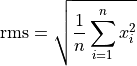The User Defined Functions chapter describes how user functions are defined.

## Channels with Missing Values¶

The formula interpreter can handle missing values.

In the following figure, channel EngSpd 6th measurement does not contain a valid value. Instead the word "Missing" is displayed.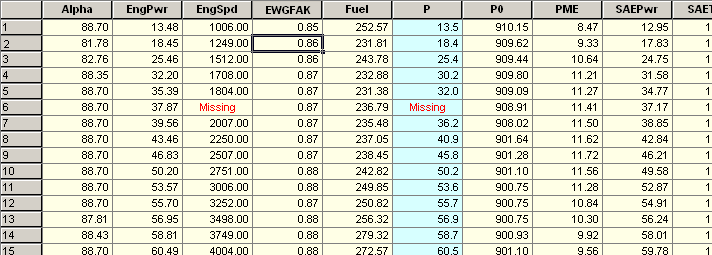Because the channel P=f(EngSpd, SAETrq) is dependant on EngSpd and the resulting channel P displays "Missing" in record 6.

MISSING_FLOAT = 1e10

## Formula Import from an Excel Workbook¶

New formulas can be specified by adding the formula to an Excel Sheet. An example file can be found in the directory UniPlot\samples. The file name is formula.xls. This file should only be used as a template. It will be overwritten the next time you install an UniPlot update. Just open the file and save it under a new name in the same directory.

To import an Excel Workbook with a formula sheet, choose Tools=>Import Formulas from Excel File.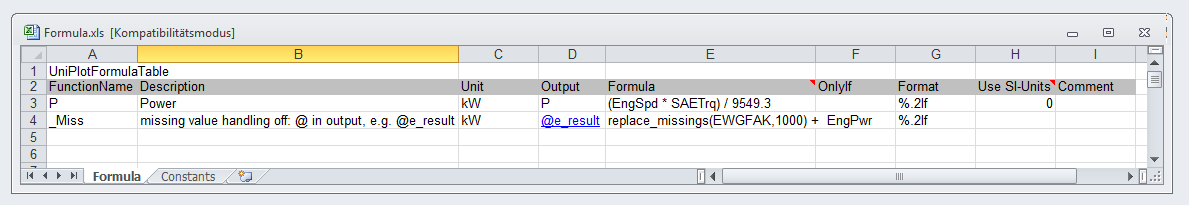The table contains 8 columns. The columns have the following meaning:

1. Column - FunctionName The formula name.
2. Column - Description A brief description.
3. Column - Unit: The formula unit. The formula interpreter only converts the formula result to the specified unit, if the SI-Units are set in column 8. Otherwise the specified unit is used only as a channel unit string.
4. Column - ChannelName: Name of the channel in the NC file.
5. Column - Formula: The formula. Example: (speed * torque) / 9549.3.
6. Column - OnlyIf: Name of a condition.
7. Column - Numberformat: Format description, e.g. %g or %lf.
8. Column - SI-Units: If set to the value 1, the variables will be converted to SI units, before the formula is evaluated. The result will be converted to the specified unit (column 3).
9. Column - Comment: This column can contain a comment. With UniPlot R2013.11, the Comment column moved to column 9. Before it was column 8.

The first cell (A1) in the Excel table must contain the key word “UniPlotFormulaTable” or “UniPlotFormelTabelle”.

The second row contains the column names.

More details about formulas can be found in chapter Formula Interpreter 2 - Creating a formula table.

id-202804